Calculus integration problem. please help. - Printable Version -Shoutbox (https://shoutbox.menthix.net) +-- Forum: MsgHelp Archive (/forumdisplay.php?fid=58) +--- Forum: General (/forumdisplay.php?fid=11) +---- Forum: General Chit Chat (/forumdisplay.php?fid=14) +----- Thread: Calculus integration problem. please help. (/showthread.php?tid=67263) Calculus integration problem. please help. by Rubber Stamp on 10-13-2006 at 11:11 AM can someone please solve this integration problem for me? i have been trying it since a week, gone mad trying to solve it. please help. (ps: its solvable. answer exists.)edit: "x.x" means x square. RE: Calculus integration problem. please help. by Rubber Stamp on 10-14-2006 at 06:11 PM could some one do it? plz reply. RE: Calculus integration problem. please help. by andrewdodd13 on 10-14-2006 at 07:58 PM Well... the square root of x^2 1/x^2 is just x + 1/x isn't it? So...RE: RE: Calculus integration problem. please help. by J-Thread on 10-14-2006 at 08:07 PM quote:Originally posted by andrewdodd13 Well... the square root of x^2 1/x^2 is just x + 1/x isn't it? No it isn't... The square root of x * x = x... So the square root of x * x + a, where a > 0, is greater then x... Right? When x > 0, 1 / (x * x) > 0, so the square root will be greater then x. You see? The integral of that formula is hard to find... and it's been a while since I have been integrating formulas... RE: Calculus integration problem. please help. by markee on 10-15-2006 at 02:01 AM Ok well I'm quite sure you have to use integration by parts. I will update this post when I get the answer for you. EDIT: I had a go and it was terribly off, probably some little mistake somewhere.  Anyway instead of trying again I used Maple and it only gave me: 2*(x^3+1/x+x/(x^4+1))^(3/2)/(3*x^2-1/x^2+1/(x^4+1)-4*x^4/(x^4+1)^2) That was after a bit of work of course.  Seemed it missed being able to do a reverse chain rule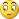.  I tried simplifying it but I couldn't find anything, maybe someone else can help.  I hope this helps you.  RE: Calculus integration problem. please help. by John Anderton on 10-15-2006 at 06:44 AM quote:Originally posted by andrewdodd13 Well... the square root of x^2 1/x^2 is just x + 1/x isn't it?wtf?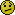If that were true most of the maths problems in the world would be solved in a few steps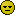RE: Calculus integration problem. please help. by Rubber Stamp on 10-15-2006 at 07:27 AM i dont think this can be done by parts. i tried using it, but the expression we get inside the second integration sign is even more complicated than the question itself. i tried substituting different forms of the expression, that hasnt helped me yet. i tried adding and substrating different terms like (x^3+1/x^3) to get some sort of differentiable term, but with not much success. i even tried substituting trigonometric properties, but that doesnt help either. RE: Calculus integration problem. please help. by Chrono on 10-15-2006 at 08:43 AM have you tried changing variables like sen( u) = x ? Or maybe the good old Tan(x/2) ? i dunno it just looks like it could be possible with one of those, im too lazy to try now though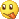maybe tomorrow RE: Calculus integration problem. please help. by John Anderton on 10-15-2006 at 01:46 PM quote:Originally posted by Chrono have you tried changing variables like sen( u) = x sin wont do it. Bleh it could but i cba to work with (sin(u))^4quote:Originally posted by Chrono Or maybe the good old Tan(x/2) ?Isnt that for places where you have trignometrics in the numerator and denominator? I was thinking of something like x^2 = (sin(u))^2 or something like that. cba atm. mabbe after my examsRE: Calculus integration problem. please help. by Rubber Stamp on 10-15-2006 at 04:22 PM nups, i already tried various trigo properties, none work. RE: Calculus integration problem. please help. by J-Thread on 10-15-2006 at 08:08 PM How do you know it is solvable?RE: Calculus integration problem. please help. by Chrono on 10-15-2006 at 08:51 PM quote:Originally posted by John Anderton x^2 = (sin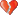)^2 lol thats the same than x=senRE: RE: Calculus integration problem. please help. by andrewdodd13 on 10-15-2006 at 09:43 PM quote:Originally posted by John Anderton quote:Originally posted by andrewdodd13 Well... the square root of x^2 1/x^2 is just x + 1/x isn't it?wtf?If that were true most of the maths problems in the world would be solved in a few stepsYeah, wasn't really thinking straight when I wrote that... I used an on-line integrator (its the first hit for "Online integrator" on google) and it gave (3x^4 - 1) ^-1 . I don't think it's right - doesn't differentiate to the correct result as far as I can tell: - (12x^3) / ((3x^4)^2) I'll have another bash it via substitution, but I think integration by parts is the way to go... but I can't do that yet. ^_^. RE: Calculus integration problem. please help. by markee on 10-15-2006 at 11:29 PM quote:Originally posted by Chrono quote:Originally posted by John Anderton x^2 = (sin)^2 lol thats the same than x=senI think he was thinking of doing something with De'Mouive's but I don't think it will work in this case.  What I did to get as far as I did was I had to first expand the equations and then use a reversal of the product rule and you should then try to simplify what you get.  It is the only way I can think of doing this problem. EDIT:  Just expanding out the equation I got it to    x^3+1/x+x/(x^4+1)    you should be able to integrate it in parts now. for some reason maple is giving me 1/4*x^4+ln(x)+1/2*arctan(x^2)  but I didn't think the arctan was right, I thought it was meant to be a ln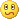and for some reason it is different to my other expressionI guess it might be that I messed up somewhere last time, I could get rid of the squareroot which i couldn't before. I don't know, lets hope I'm not as dodgy in my maths tutorial this afternoon, I'll ask my teacher to help if I rememberRE: Calculus integration problem. please help. by Chrono on 10-16-2006 at 12:29 AM ah maple, im glad i dont need to use that thing anymore. i really disliked that program for some reason, even though it was pretty useful for Diferential equationsRE: Calculus integration problem. please help. by Rubber Stamp on 10-17-2006 at 05:23 PM quote:Originally posted by J-Thread How do you know it is solvable?  its from a set of question from a pretty respectable book. RE: Calculus integration problem. please help. by kotjze on 10-17-2006 at 05:41 PM quote:Originally posted by markee EDIT:  Just expanding out the equation I got it to    x^3+1/x+x/(x^4+1)    you should be able to integrate it in parts now. for some reason maple is giving me 1/4*x^4+ln(x)+1/2*arctan(x^2) x^3+1/x+x/(x^4+1) does not equal (x+1/x)*sqrt(x^2+1/x^2) which is why maple gave you the wrong answer. RE: RE: RE: Calculus integration problem. please help. by Jesus on 10-17-2006 at 06:13 PM quote:Originally posted by andrewdodd13 I used an on-line integrator (its the first hit for "Online integrator" on google) and it gave (3x^4 - 1) ^-1 . I don't think it's right - doesn't differentiate to the correct result as far as I can tell: - (12x^3) / ((3x^4)^2)lol I did that too, got this:don't know if it's correct, looks far too complicated for meedit: image is gone, will try to get it back edit2: got it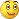RE: Calculus integration problem. please help. by s7a5 on 10-17-2006 at 09:18 PM never did this type of problem b4, and substitution technique doesnt seem to work for it so maybe that's how it goes: we know that : (x^2 + 1/x^2)^1/2 = (x + 1/x) so we are left with: S (x + 1/x) (x + 1/x) multiply them we get: S (x^2 + 2 + x^-2) so the answer is: 1/3 x^3 + 2x - 1/x Again Im not sure and didnt look for other ways to solve it, but who knows maybe I'm right RE: Calculus integration problem. please help. by kotjze on 10-18-2006 at 01:50 AM quote:Originally posted by s7a5 never did this type of problem b4, and substitution technique doesnt seem to work for it so maybe that's how it goes: we know that : (x^2 + 1/x^2)^1/2 = (x + 1/x) Really? So... (5^2 + 1/6^2)^1/2 = (5 + 1/6)? No. If that were true, then (x + 1/x)^2 = (x^2 + 1/x^2), but if you work that out, it's (x^2 + 2 + 1/x^2) RE: Calculus integration problem. please help. by Vilkku on 10-18-2006 at 04:54 AM I don't have enough time to actually to solve it atm, but I think I know how I would attempt it. int*(x + 1/x) * int*((x^2 + 1/x^2)^(1/2)) As I'm sure someone pointed out earlier. 1/x^2 indeed is 1/x. Try it with a number and put it in your calculator. Insttead of agruing about the square root part, why don't just fuse the expressions under the root to one? Might be more complicated, but at least you can't have different opinions then. x^2 + 1/x^2 = (x^2 + 1) / x^2 Now of course, we will have the fun of realising there are no x outside the parenthesis, so we will have to divide and get some funky number, which I'm certaninly not arsed to do atm. EDIT: As I wasn't sure about the thing you are arguing on, I decided just to comment it. Shouldn't it be possible just to take the square root of x^2, which is x, and the root of 1/x^2, which is 1/x, and then write it as x + 1/x and then int* that part? RE: Calculus integration problem. please help. by Chrono on 10-18-2006 at 05:01 AM quote:Originally posted by Vilkku int*(x + 1/x) * int*((x^2 + 1/x^2)^(1/2))you cant do that, lolcounterexample: int x^2 =(X^3)/3 int x * int x = (x^4)/4 i tried to solve it earlier but didnt manage to get to an answer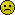i used to be good at those, but it's been a long time since i had to integrate a difficult expressionquote:Originally posted by Vilkku EDIT: As I wasn't sure about the thing you are arguing on, I decided just to comment it. Shouldn't it be possible just to take the square root of x^2, which is x, and the root of 1/x^2, which is 1/x, and then write it as x + 1/x and then int* that part? ew that's so wrong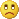you cant do that either... RE: RE: Calculus integration problem. please help. by Vilkku on 10-18-2006 at 05:02 AM quote:Originally posted by Chrono quote:Originally posted by Vilkku int*(x + 1/x) * int*((x^2 + 1/x^2)^(1/2))you cant do that, lolcounterexample: int x^2 =(X^3)/3 int x * int x = (x^4)/4 i tried to solve it earlier but didnt manage to get to an answeri used to be good at those, but it's been a long time since i had to integrate a difficult expressionOh yeah, it has to be + or - between them... was too long scince I did this. RE: RE: Calculus integration problem. please help. by J-Thread on 10-18-2006 at 11:55 AM quote:Originally posted by Rubber Stamp quote:Originally posted by J-Thread How do you know it is solvable?  its from a set of question from a pretty respectable book. I've got a pretty respectable book that asks if P=NP, but I didn't manage to solve that either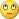However, I think I have enough mathematical knowledge to solve this problem, but as I stated before, it a pretty tough one. A lot of people have failed... But when I have some spare time I will try to solve it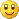RE: RE: Calculus integration problem. please help. by andrewdodd13 on 10-18-2006 at 10:50 PM quote:Originally posted by s7a5 never did this type of problem b4, and substitution technique doesnt seem to work for it so maybe that's how it goes: we know that : (x^2 + 1/x^2)^1/2 = (x + 1/x) so we are left with: S (x + 1/x) (x + 1/x) multiply them we get: S (x^2 + 2 + x^-2) so the answer is: 1/3 x^3 + 2x - 1/x Again Im not sure and didnt look for other ways to solve it, but who knows maybe I'm right That's what I done.. (x^2 + 1/x^2) ^ 1/2 != (x+1/x). Via parts, I get the following...I don't think it's right, I cba differentiating it atm, because I'd then have to do something fancy to get it into its original form. However, I also don't think by parts will work because of the sqrt... RE: Calculus integration problem. please help. by markee on 10-18-2006 at 11:45 PM You sure the question wasn't (x + 1/x)(x^2 + 2 + 1/(x^2))^(1/2) ? if that was the case then it would be simply the integral of (x + 1/x)^2 but without that +s under the quareroot it makes it almightily difficult.  Or even if (x + 1/x) was replaced with the derivative of (x^2 + 1/(x^2)).  I'll keep trying my best to get an answer, I got something at home but it's a bit ugly and I have to check with it's derivative just to make sure I did it right but I didn't want to just yet because of it's uglinessRE: Calculus integration problem. please help. by J-Thread on 10-21-2006 at 05:52 AM I tried and I thought I had the good idea, but I came this far (see attachement). Now I'm stuck again...it is really a difficult one! RE: Calculus integration problem. please help. by Chrono on 10-21-2006 at 05:59 AM haha i did the exact same thing, that was actually my first try. The file's nice, but you did way too many steps. You could have done it in three lines. it's too tedious to read all thatRE: Calculus integration problem. please help. by markee on 10-21-2006 at 02:27 PM I did the same thing in about as many steps as chrono.  Will post my steps from there. EDIT:  Here are my steps to follow on... code:dy/dx = int( (x^4 + 2x^2 + 2 + 2/x^2 + 1/x^4)^1/2 )   what you had y = (x^4 + 2x^2 + 2 + 2/x^2 + 1/x^4)^3/2              reversal of the chain rule      3/2 * (4x^3 + 4x - 4/x^3 - 4/x^4) y = (x^4 + 2x^2 + 2 + 2/x^2 + 1/x^4)^3/2             simplified somewhat      6 * (x^3 + x - 1/x^3 - 1/x^4 see why I wanted to simplify it a bit..... RE: RE: Calculus integration problem. please help. by J-Thread on 10-22-2006 at 12:05 PM quote:Originally posted by Chrono The file's nice, but you did way too many steps. You could have done it in three lines. it's too tedious to read all thatThat's just what you like or not. I am used to writing my steps down as clear as possible. Especially when I am proving something, I like to do easy steps that everybody can check. If no step is wrong, I have proved the fact I wanted to prove. When I do "bigger" steps, the prove will be a bit hard to follow. Of course I see the bigger steps myself, but I know that not everybody does. However, it seems like this trick also didn't work. I honestly begin to believe that there isn't a solution to this problem, but I can't prove it yet. And I guess it would be easier to solve a problem (if a solution exists) then proving that no solution exists... RE: Calculus integration problem. please help. by Buttah N Bred on 02-15-2007 at 12:19 PM Wow!  It appears that everyone is getting something different.  Here is what I did.  I used substitution and realized that the 1/x^2 can be rewritten as 1x^-2. Integration: (x + 1/x) (sqrroot(x^2+x^-2)) dx u = x^2+x^-2 dx du = 2x - 2x^-1 dx (1/2)du = x - x^-1 x- x^-1 = x + 1/x [color=black] Substitute (x + 1/x) with 1/2 and x^2+x^-2 with "u" Integrate from here: 1/2 (u^(1/2)) 1/2 (u^(3/2)) / (3/2)       2u^(3/2)         2 * 3 this equals: 2(x^2 + 1/x^2)^(3/2)                                  6 THIS WAS CONFIRMED BY A TI-89 Titanium.  Check it yourself.  No integration by parts neccessary! RE: Calculus integration problem. please help. by Rolando on 02-15-2007 at 01:04 PM quote:Originally posted by Buttah N Bred Wow!  It appears that everyone is getting something different.  Here is what I did.  I used substitution and realized that the 1/x^2 can be rewritten as 1x^-2. Integration: (x + 1/x) (sqrroot(x^2+x^-2)) dx u = x^2+x^-2 dx du = 2x - 2x^-1 dx (1/2)du = x - x^-1 x- x^-1 = x + 1/x [color=black] Substitute (x + 1/x) with 1/2 and x^2+x^-2 with "u" Integrate from here: 1/2 (u^(1/2)) 1/2 (u^(3/2)) / (3/2)       2u^(3/2)         2 * 3 this equals: 2(x^2 + 1/x^2)^(3/2)                                  6 THIS WAS CONFIRMED BY A TI-89 Titanium.  Check it yourself.  No integration by parts neccessary! Nice math but.. did you see the date of the other posts?Months ago. RE: Calculus integration problem. please help. by Buttah N Bred on 02-15-2007 at 08:17 PM no,  I wasn't focused on the date that it was posted.  this was for anybody else who may have had this problem.